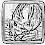## Friday, June 05, 2009

### Triangles Optical Illusion

1.how can a triange with sides of the ratio 2:3 be similar to one that has sides of the ratio of 8:3

2.You have to take the area of all the blocks and not just one block.

First triangle is formed by using few blocks. The same blocks are realigned and formed second triangle. But, in the second triangle, there is one empty square. Why is it so? That is the question.

3.thats what i am saying... its not a triangle... its a quadilatral... the hypotenuse of the so called larger triangle is not a straight line

4.Your answer is correct. I got confused because of the ration no.s that you specified. I could not understand what they map to.

They are not triangles. For it to be a triangle, both the red and dark green triangles should be similar. But, in this case, one is 2:5, and another one is 3:8. For it to be a triangle, it has to be 3:7.5.

5.who invented this triangle?

6.7.volumes aren't additive, everybody knows this

8.9.The easiest way of seeing this is to look at where the red and blue triangles meat. In the top they meat at 8,3 in the bottom that's not on a line.

10.i dont understand. everyone is saying that the bottom triangle doesnt have a straight hypotenuse, because of the side ratio of the two smaller trianges. BUT i put a ruler to it and they form a perfect straight line, if its a quadrilatteral, the the fourth angle bust be like 179.5 or something

1.You need a better ruler for a start. Also the uneven thickness of the outline of the "triangles" is designed to throw you off, as are the different colours. Easiest way would be to get some gridded paper, plot the points that you see on it and then overlay a triangle with only the three corner points. You'll see that the hypotenuse of the top "triangle" is actually concave and that of the bottom "triangle" is convex.

Alternatively, you should be able work out that if the hypotenuse of the triangle is a straight line, then any triangles formed off it should have the same ratios for the opposing and adjacent sides, but they don't here. Using the ratios given and basic trigonometry, the green triangle has internal angles 90, 21.8 and 68.2, the red has 90, 20.6 and 69.4. As a result, where red meets green in the top shape, the "line" actually has an internal angle of 181.2, making it concave; similarly where red meets green in the lower shape the "line" has an internal angle of 178.1, giving a convex shape.

You don't actually need to go through all that anyway, given that if you look closely enough, you can see the blatant distortion of the shapes as they are, compared to an actual triangle.

11.It is difficult to do find it by using ruler, because, there would be some error, when we do that way. We need to do it mathematically.

12.First picture 5*13/2=65/2=32,5
Second picture we don't have complete triangle so we ll do each figure
4*3/2=12
2*5/2=5
2*2+1*3=7
2*3+2*1=8
second picture = 32
So the diference is 0,5 . But in picture we see 1.
This should be optical illusion so we need to look close.
Look in every square and sea the border area that triangle covers , if you look close you can notice that picture 1 and picture 2 are not the same.